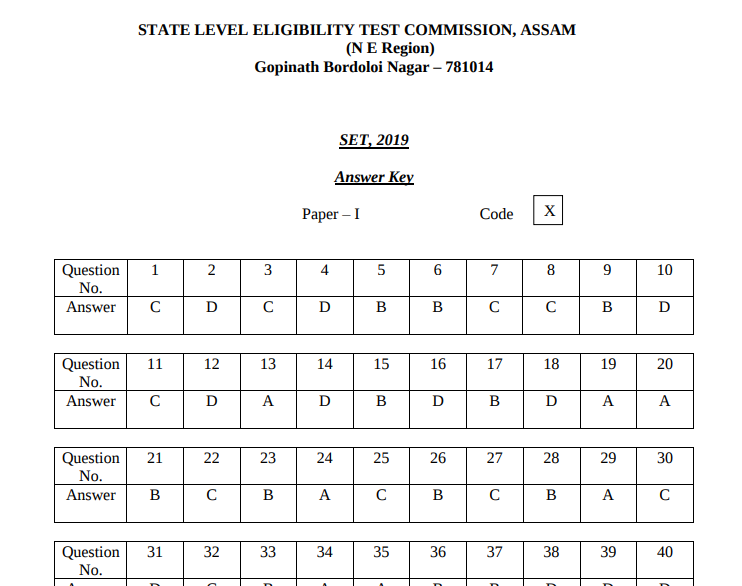Initially, the commission will release the provisional SET answer key 2020. In case of discrepancies, candidates will be provided with the facility to raise objections.The final answer key of SET 2020 will be released after evaluating the objections submitted by the candidates. For more details about SET answer key 2020, read the article below.

Latest: To check SET Result 2019 here

## SET Answer Key 2020 Dates

Candidates can check the exam as well as SLET answer key dates from the table provided below.

 Events Dates NESLET exam March 2020 SET answer key 2020 (provisional) To be notified SET final answer key 2020 To be notified

Step 1: Go to the official website - sletne.org

Step 2: Click on the link “SET/SLET answer key’ to be provided

Step 3: Select the test booklet set

Step 4: The answer key pdf appears on the screen.

Step 6: Check the responses

Step 7: Calculate your probable score

### Answer Key of SET will look like the image provided below:The exam conducting body initially releases the provisional answer key of SET 2020. The answer key will be available in the form of PDF for both the papers and all the sets. Candidates can also raise objections in case they find discrepancies in the SET 2020 answer key. They can check and download the last year’s provisional answer key from the table given below:

### What to do in case of any discrepancy in the SET answer key 2020?

In case candidates find discrepancies in the SET 2020 answer key, then they can raise objections. In order to raise objections, candidates have to contact the exam conducting authority mentioning details such as the exam name, paper appeared for, question booklet series, question number, and explanation for the objection till the prescribed day.

### Revised SET Answer Key 2020

After evaluating the objections submitted by the candidates, the exam conducting body will release the final/ revised answer key of SET 2020. The commission decision on the challenges shall be final and the result will be declared on the basis of final answer key. Candidates can check below last year’s SET answer key from the table given below.

### SET Answer Key 2019 - Final

Due to a typographical mistake in question no. 15 series X, question no. 30 series Y, and question no 48 Series Z, the earlier published final/revised answer key of SET was replaced. Check below the final answer key of SET.

### SET Answer 2020 Key - Calculation of Score

In order to calculate the marks, candidates will have to go through the exam pattern to know the marking scheme. The exam of SLET is conducted for two papers – paper I and II. Both Paper I and II will be allotted 2 marks for each correct answers. As there will not be any negative marking, candidates will be able to calculate their expected marks based on the number of correct answers they will give. The formula to calculate SET Marks:

 Total Marks Obtained = Number of correct answers – Marks for wrong answers

### Candidates can check the steps given below to calculate their expected score.

• Calculate all the number of correct answers and allocate the marks accordingly

• Now, count the number of incorrect answers

• Now, calculate the total marks secured for correct answer and candidates will know their probable score.

### FAQs related to SET Answer Key 2020

Question: What is the marking scheme of SLET/SET?
Answer: As per the marking scheme of SET, each correct answer will be awarded 2 marks. There will be no negative marking for incorrect responses. The formula to calculate SET marks is - Score = Number of correct responses – Number of incorrect answers.

Question: What are the benefits of SET 2020 Answer Key?
Answer: With the help of SET answer key 2020, can get all the correct answers to the questions asked in the exam. Apart from this, candidates will also be able to calculate their probable score.

Question: What to do if there is any discrepancy in the answer key of SET?
Answer: In case of discrepancies, you need to contact the exam conducting body and provide details such as the exam name, paper appeared for, question booklet series, question number, and explanation for the objection till the prescribed day.

Question: Will the exam conducting body release the revised SET answer key 2020?
Answer: After reviewing the provisional answer key of SET, the commission will release the final answer key.

Question: When will SET answer key 2020 be released?
Answer: The answer key of SET is released after seven days from the date of exam.

### Applications Open NowIELTS
View All Application Forms

## More like SET (SLET)

Top
The question have been saved in answer later, you can access it from your profile anytime. Access now